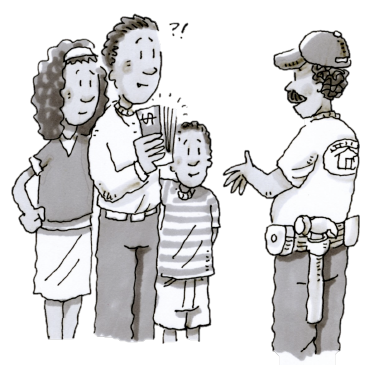### Home > CCAA > Chapter 7 Unit 6 > Lesson CC2: 7.1.8 > Problem7-91

7-91.

The Jones family wants to remodel their kitchen. They have saved $\23,000$ in the last two years. Their contractor says the remodel will cost $\40,000$. They can borrow the difference at a monthly interest rate of $2\%$ simple interest. If they pay the loan off in six months, how much will they have paid?

First calculate how much money the Jones need to borrow.
$\40,000-\23,000=\17,000$

Calculate the amount of interest that is added per month.
$\17,000(0.02)=\340$

Since the Jones can pay off the loan in $6$ months, multiply the amount of interest added per month by $6$.
$\340(6)=\2,040$

Finally, add that amount to the original to find the amount of money the Jones need to pay.
$\17,000+\2,040=\19,040$

Do you see how the simple interest formula was used?
$I=(17,000)(0.02)(6)$